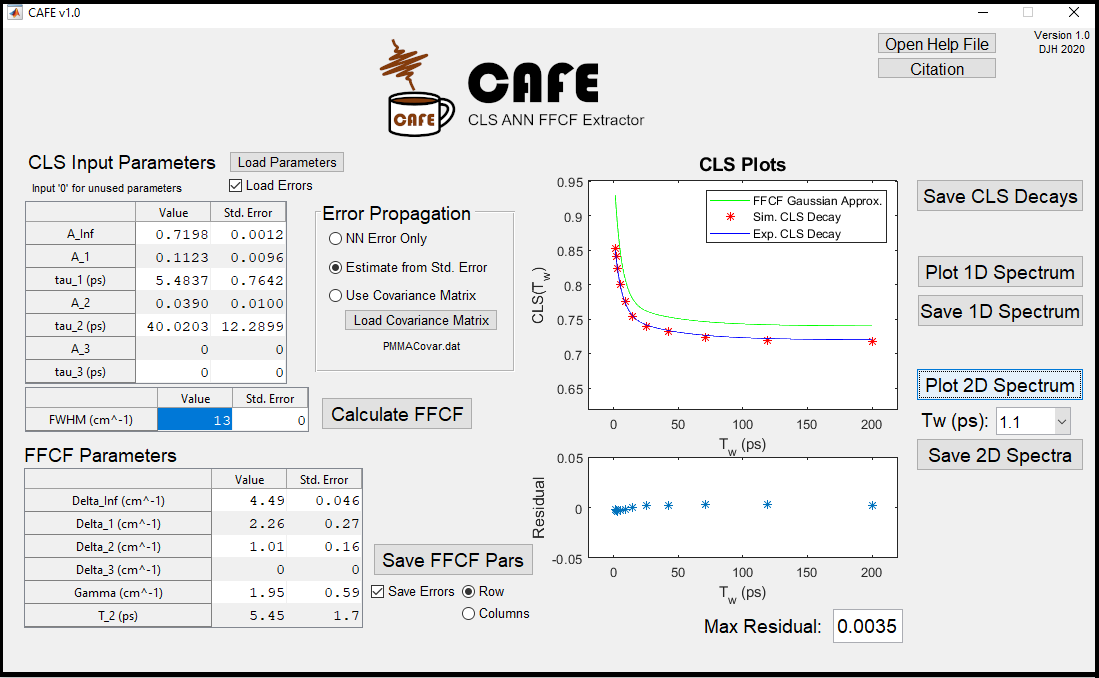CAFE is software used to process the results of the Center Line Slope (CLS) analysis that is used as an observable of spectral diffusion in 2D correlation spectroscopy to obtain the underlying time-correlation function, the Frequency-Frequency Correlation Function. The program uses the theoretical relationships between the CLS and FFCF as well as artificial neural networks (ANNs) to quickly and accurately calculate the parameterized FFCF from a parameterized CLS decay and linear absorption spectrum. Complete details of the underlying algorithm can be found in the associated publication and SI documents (1 (PDF) | 2 (XLSX) ). CAFE is currently freely available in two forms: a complete standalone GUI-based program and a core MATLAB library that can be run from the MATLAB command line or integrated into other MATLAB software.

## Version History

v.1.1 (March 2021):

• Added automatic error correction algorithm for "Calculated linewidth exceeds experimental FWHM" error
• (GUI Only) Added table font size selection

# CAFE GUIThe CAFE GUI is presently only available for Windows 64-bit operating systems and requires installation of the free MATLAB Runtime Environment (MRE) v. 9.7. CAFE GUI does not require a full MATLAB installation on the same device. Additional MATLAB software files are available upon request.

CAFE GUI provides:

• Calculation of the FFCF parameters from CLS decay parameters
• Error propagation of FFCF parameters from CLS parameter covariance
• Calculated 1D and 2D spectra based on the calculated FFCF
• The CLS values for the calculated 2D spectra and the FFCF Gaussian approximation

# CAFE MATLAB Library

The CAFE MATLAB library has been confirmed to successfully run on MATLAB releases between 2014b and 2019b.

CAFE Library provides:

• Calculation of the FFCF parameters from CLS decay parameters
• Error propagation of FFCF parameters from CLS parameter covariance
• Calculated 1D spectrum based on the calculated FFCF
• Command line tools that allow for easy incorporation into other MATLAB software